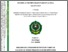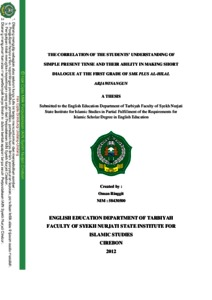# THE CORRELATION OF THE STUDENTS’ UNDERSTANDING OF SIMPLE PRESENT TENSE AND THEIR ABILITY IN MAKING SHORT DIALOGUE AT THE FIRST GRADE OF SMK PLUS AL-HILAL ARJAWINANGUN

OMAN RINGGIT, (2012) THE CORRELATION OF THE STUDENTS’ UNDERSTANDING OF SIMPLE PRESENT TENSE AND THEIR ABILITY IN MAKING SHORT DIALOGUE AT THE FIRST GRADE OF SMK PLUS AL-HILAL ARJAWINANGUN. Bachelor thesis, IAIN Syekh Nurjati Cirebon.Preview
Text
OMAN RINGGIT_58430500__OK.pdf

Download (986kB) | Preview

## Abstract

OMAN RINGGIT “The Correlation of the Students’ Understanding of Simple Present Tense and Their Ability in Making Short Dialogue at the First Grade of SMK Plus Al Hilal Arjawinangun”. Simple present tense is the first basic tense that should mastered by students in senior high school grade. Before students understand the other tenses or other grammar, students should be able to understand simple present tense first. Because understanding about English can help students in understanding about the next grammar. How about making short dialogue? Do students need to understand simple present tense first in making short dialogue? Making short dialogue is the simplest and the most efficient in practicing English. It’s very important to develop their ability in English. The aims of the research are to find out the students’ understanding of simple present tense, to find out the students’ ability in making short dialogue and to find out if there is a significance correlation of the students’ understanding of simple present tense and their ability in making short dialogue The approach of the research in writing this thesis is a quantitative approach, it is correlation research. It means that data which is obtained from the field of research then analyzed statically. The Pearson’s product moment correlation formulation, the formula of t and the formula for degree of freedom (dF) was used to compute the correlation between two variables. The population of the research is all of students at the first grade of SMK Plus Al Hilal Arjawinangun. All of students at first grade consist of one class. In the research the writer took 100 % of the Population. The analysis of the test shows that the average score of the students’ test of simple present tense was 70 and the average score of the students’ test in making short dialogue was 78. Both of these score can be categorized in good. Meanwhile, the calculation by using Product Moment Correlation by Pearson, indicates the result of the correlation between the students’ understanding of simple present tense and their ability making short dialogue is 0.37, its mean that the between X and Y variable has weak correlation. In other words, there is a positive influence of the student’s understanding of simple present tense on their ability in making short dialogue at the first grade of SMK Plus Al Hilal Arjawinangun.

Item Type: Thesis (Bachelor) Pendidikan > Pendidikan (Umum) tuti alawiyah alawiyah 22 Mar 2017 06:10 07 Jun 2017 01:32 http://repository.syekhnurjati.ac.id/id/eprint/1251

### Actions (login required)View Item